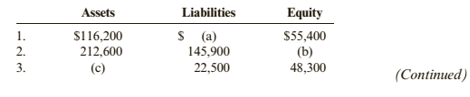Chapter 1, Problem 36E### Cornerstones of Financial Accounti...

4th Edition
Jay Rich + 1 other
ISBN: 9781337690881

#### Solutions

Chapter
Section### Cornerstones of Financial Accounti...

4th Edition
Jay Rich + 1 other
ISBN: 9781337690881
Textbook Problem

# Exercise 1-36 The Fundamental Accounting EquationFinancial information for three independent cases is given belowRequired:Compute the missing numbers in each case.

To determine

Concept Introduction:

Accounting equation:

Accounting equation represents the mathematical relationship between assets, liabilities and equity. According to this equation, assets are equal to the sum of liabilities and equity. The formal for basic accounting equation is as follows:

Assets = Liabilities + Equity

To Calculate:

The missing amounts using the accounting equation.

Explanation

The missing amounts are calculated as follows:

 Case Assets Liabilities Equity 1 $116,200$ 60,800 \$55,400 (116200-55400)

### Still sussing out bartleby?

Check out a sample textbook solution.

See a sample solution

#### The Solution to Your Study Problems

Bartleby provides explanations to thousands of textbook problems written by our experts, many with advanced degrees!

Get Started

#### What is logical independence?

Database Systems: Design, Implementation, & Management

#### List two applications of Acme threads.

Precision Machining Technology (MindTap Course List)

#### What is outsourcing? How is it different from offshoring?

Principles of Information Systems (MindTap Course List)